NCERT Solutions for Class 8 Maths Chapter 11 Mensuration Ex 11.1 are part of NCERT Solutions for Class 8 Maths. Here we have given NCERT Solutions for Class 8 Maths Chapter 11 Mensuration Ex 11.1.

 Board CBSE Textbook NCERT Class Class 8 Subject Maths Chapter Chapter 11 Chapter Name Mensuration Exercise Ex 11.1, Ex 11.2, Ex 11.3, Ex 11.4 Number of Questions Solved 5 Category NCERT Solutions

## NCERT Solutions for Class 8 Maths Chapter 11 Mensuration Ex 11.1

Question 1.
A square and a rectangular field with measurements as given in the figure have the same perimeter. Which field has a larger area?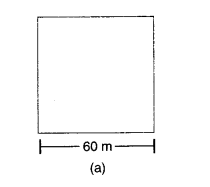Solution.
Area of the square field = a x a
= 60 m x 60 m = 3,600 $${ m }^{ 2 }$$
Perimeter of the square field = 4a
= 4 x 60 m = 240 m
∴ Perimeter of rectangular field = 240 m
⇒ 2(l + b) = 240
⇒ 2(80 + b) = 240
where b m is the breadth of the rectangular field
⇒ 80 + b = $$\frac { 240 }{ 2 }$$ ⇒ 80 + bx = 120
⇒ b = 120 – 80 = 40
∴ Area of rectangular field
= l x b = 80 m x 40 m = 3,200 $${ m }^{ 2 }$$
So, the square field (a) has a larger area

Question 2.
Mrs. Kaushik has a square plot ‘ with the measurement as shown in the figure. She wants to construct a house in the middle of the plot. A garden is developed around the house. Find the total cost of developing a: garden around the house at the rate of ₹ 55 per $${ m }^{ 2 }$$.
Solution.
Area of the square plot = a x a
= 25 x 25 $${ m }^{ 2 }$$ = 625 $${ m }^{ 2 }$$
Area of the house = a x b
= 20 x 15 $${ m }^{ 2 }$$ = 300 $${ m }^{ 2 }$$
∴ Area of the garden
= Area of the square plot – Area of the house
= 625 $${ m }^{ 2 }$$ – 300 $${ m }^{ 2 }$$
= 325 $${ m }^{ 2 }$$
∵ The cost of developing the garden per square metre = ₹ 55.
∴ Total cost of developing the garden
= ₹ 325 x 55
= ₹ 17,875.

Question 3.
The shape of a garden is rectangular in the middle and semicircular at the ends as shown in the diagram. Find the area and the perimeter of this garden.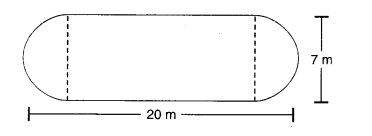Solution.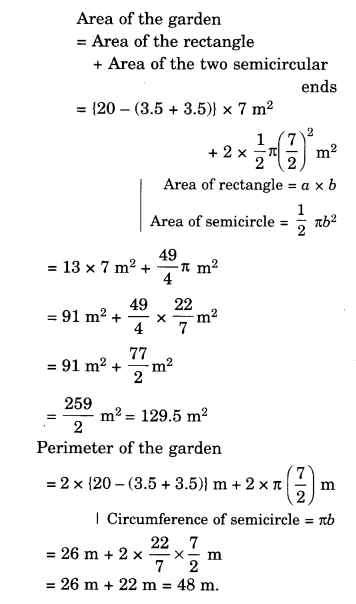Question 4.
A flooring tile has the shape of a parallelogram whose base is 24 cm and the cor-responding height is 10 cm. How many such tiles are required to cover a floor of area 1080 m2? (If required you can split the tiles in whatever way you want to fill up the corners).
Solution.
Area of a flooring tile = bh
= 24 x 10 $${ cm }^{ 2 }$$
= 240 $${ cm }^{ 2 }$$
Area of the floor
= 1080 $${ m }^{ 2 }$$
= 1080 x 100 x 100 $${ cm }^{ 2 }$$
∵ m2 = 100 x 100 $${ cm }^{ 2 }$$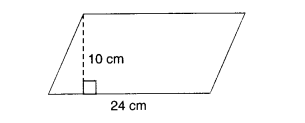∴ Number of tiles required to cover the floor
=$$\frac { Area\quad of\quad the\quad floor\quad }{ Area\quad of\quad a\quad flooring\quad tile }$$
= $$\frac { 1080\times 100\times 100\quad }{ 240 }$$
= 45000.

Question 5.
An ant is moving around a few food pieces of different shapes scattered on the floor. For which food-piece would the ant have to take a longer round? Remember, the circumference of a circle can be obtained by using the expression c = 2πr, where r is the radius of the circle.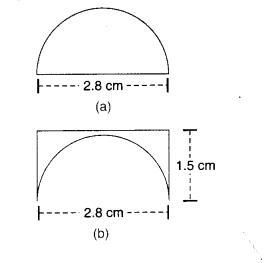Solution.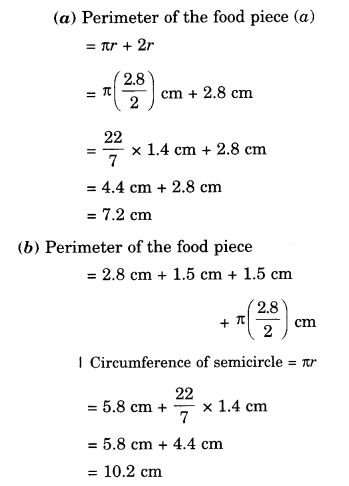We hope the NCERT Solutions for Class 8 Maths Chapter 11 Mensuration Ex 11.1 help you. If you have any query regarding NCERT Solutions for Class 8 Maths Chapter 11 Mensuration Ex 11.1, drop a comment below and we will get back to you at the earliest.